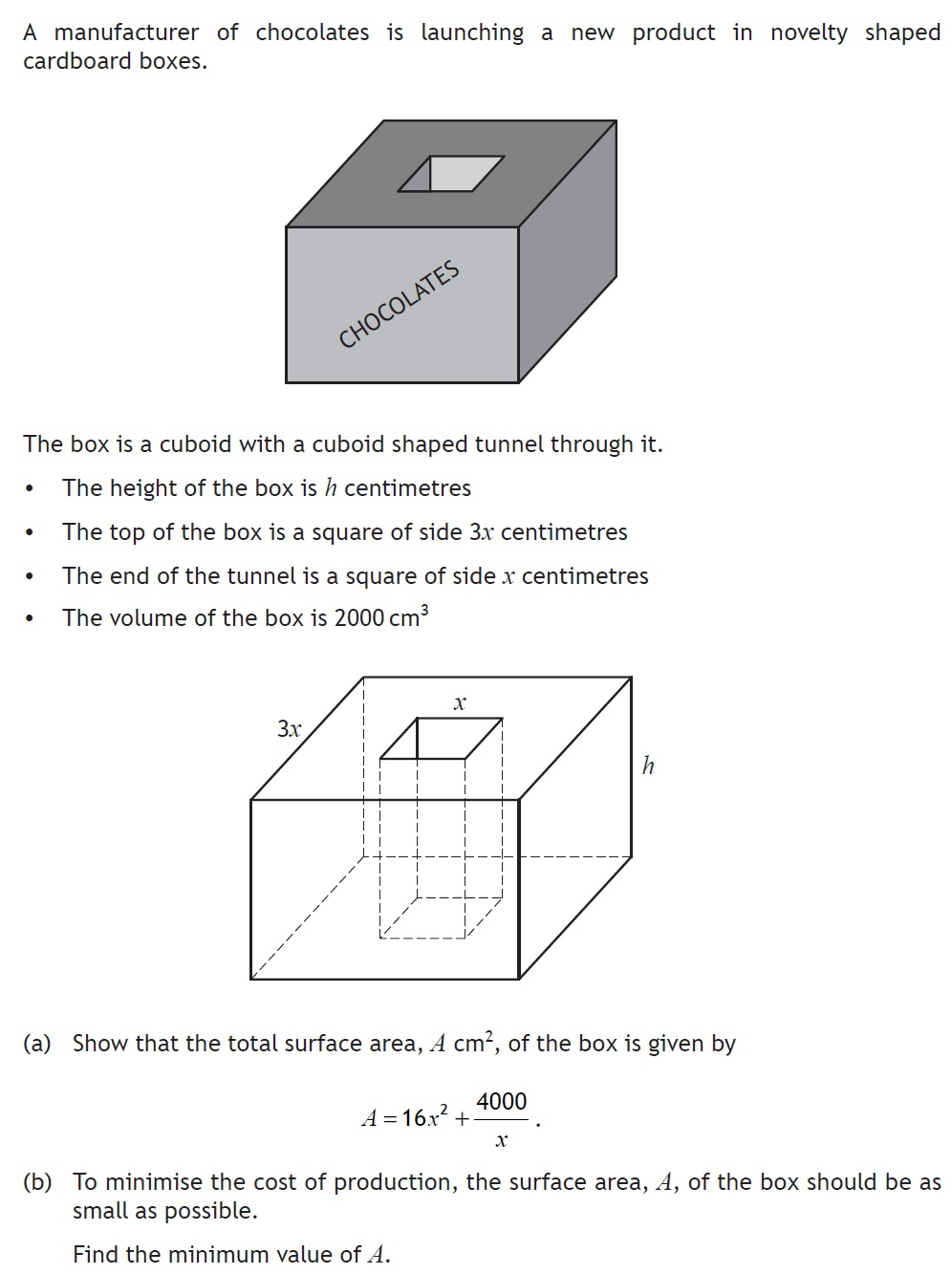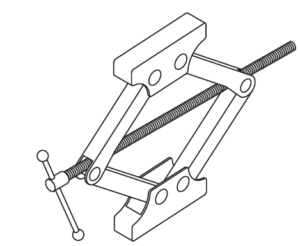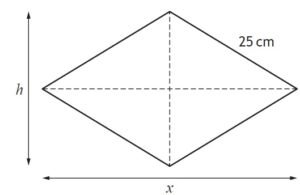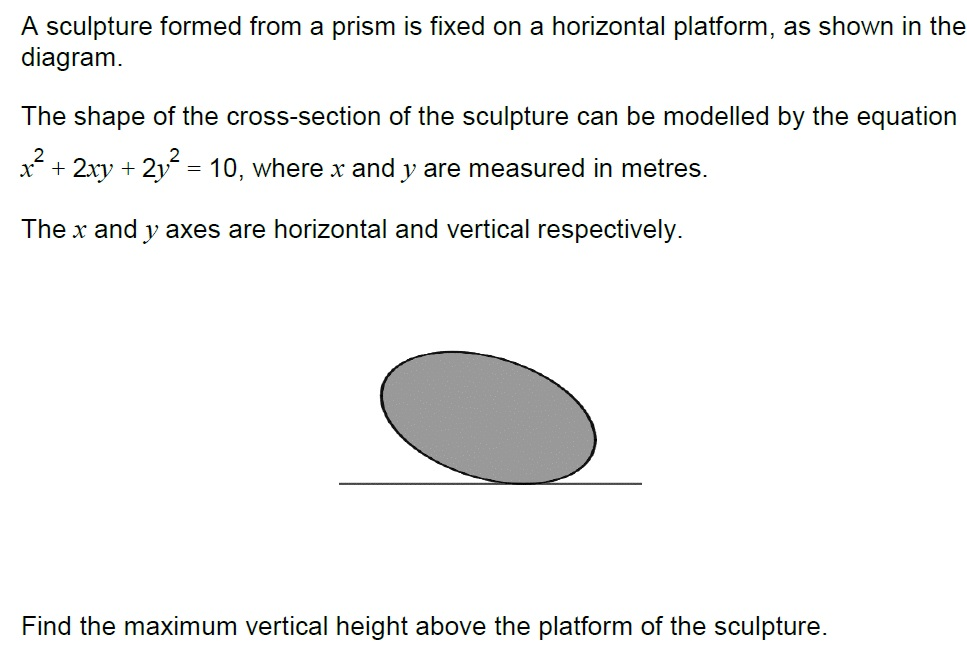# Practice Questions – Application of derivatives

Q1. [ Non calculator]2. An engineer has designed a lifting device. The handle turns a screw which shortens the horizontal length and increases the vertical height.The device is modelled by a rhombus, with each side 25 cm.
The horizontal length is  $x$ cm, and the vertical height is  $h$ cm as shown.(a) Show that  $h=\sqrt{2500–{x}^{2}}$ .    [1 mark]
(b) The horizontal length decreases at a rate of 0·3 cm per second as the handle is turned.
Find the rate of change of the vertical height when  $x=30$ .   [5 marks]

3.